# Graph Theory - Fundamentals

A graph is a diagram of points and lines connected to the points. It has at least one line joining a set of two vertices with no vertex connecting itself. The concept of graphs in graph theory stands up on some basic terms such as point, line, vertex, edge, degree of vertices, properties of graphs, etc. Here, in this chapter, we will cover these fundamentals of graph theory.

### Point

A point is a particular position in a one-dimensional, two-dimensional, or three-dimensional space. For better understanding, a point can be denoted by an alphabet. It can be represented with a dot.

### ExampleHere, the dot is a point named ‘a’.

### Line

A Line is a connection between two points. It can be represented with a solid line.

Example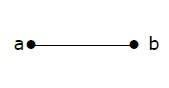Here, ‘a’ and ‘b’ are the points. The link between these two points is called a line.

### Vertex

A vertex is a point where multiple lines meet. It is also called a node. Similar to points, a vertex is also denoted by an alphabet.

Example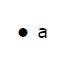Here, the vertex is named with an alphabet ‘a’.

### Edge

An edge is the mathematical term for a line that connects two vertices. Many edges can be formed from a single vertex. Without a vertex, an edge cannot be formed. There must be a starting vertex and an ending vertex for an edge.

Example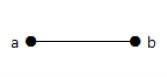Here, ‘a’ and ‘b’ are the two vertices and the link between them is called an edge.

### Graph

A graph ‘G’ is defined as G = (V, E) Where V is a set of all vertices and E is a set of all edges in the graph.

Example 1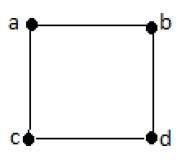In the above example, ab, ac, cd, and bd are the edges of the graph. Similarly, a, b, c, and d are the vertices of the graph.

Example 2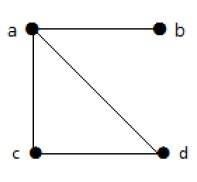In this graph, there are four vertices a, b, c, and d, and four edges ab, ac, ad, and cd.

### Loop

In a graph, if an edge is drawn from vertex to itself, it is called a loop.

Example 1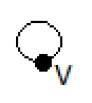In the above graph, V is a vertex for which it has an edge (V, V) forming a loop.

Example 2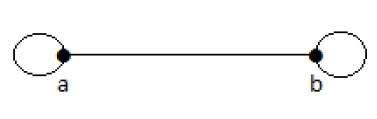In this graph, there are two loops which are formed at vertex a, and vertex b.

## Degree of Vertex

It is the number of vertices adjacent to a vertex V.

Notation − deg(V).

In a simple graph with n number of vertices, the degree of any vertices is −

deg(v) ≤ n – 1 ∀ v ∈ G

A vertex can form an edge with all other vertices except by itself. So the degree of a vertex will be up to the number of vertices in the graph minus 1. This 1 is for the self-vertex as it cannot form a loop by itself. If there is a loop at any of the vertices, then it is not a Simple Graph.

Degree of vertex can be considered under two cases of graphs −

• Undirected Graph

• Directed Graph

## Degree of Vertex in an Undirected Graph

An undirected graph has no directed edges. Consider the following examples.

Example 1

Take a look at the following graph −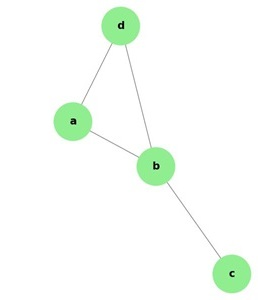In the above Undirected Graph,

• deg(a) = 2, as there are 2 edges meeting at vertex ‘a’.

• deg(b) = 3, as there are 3 edges meeting at vertex ‘b’.

• deg(c) = 1, as there is 1 edge formed at vertex ‘c’

• So ‘c’ is a pendent vertex.

• deg(d) = 2, as there are 2 edges meeting at vertex ‘d’.

• deg(e) = 0, as there are 0 edges formed at vertex ‘e’.

• So ‘e’ is an isolated vertex.

Example 2

Take a look at the following graph −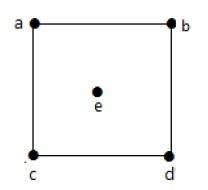In the above graph,

deg(a) = 2, deg(b) = 2, deg(c) = 2, deg(d) = 2, and deg(e) = 0.

The vertex ‘e’ is an isolated vertex. The graph does not have any pendent vertex.

## Degree of Vertex in a Directed Graph

In a directed graph, each vertex has an indegree and an outdegree.

### Indegree of a Graph

• Indegree of vertex V is the number of edges which are coming into the vertex V.

• Notation − deg−(V).

### Outdegree of a Graph

• Outdegree of vertex V is the number of edges which are going out from the vertex V.

• Notation − deg+(V).

Consider the following examples.

Example 1

Take a look at the following directed graph. Vertex ‘a’ has two edges, ‘ad’ and ‘ab’, which are going outwards. Hence its outdegree is 2. Similarly, there is an edge ‘ga’, coming towards vertex ‘a’. Hence the indegree of ‘a’ is 1.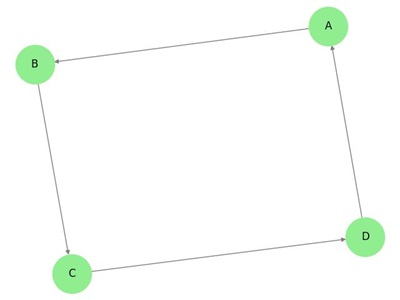The indegree and outdegree of other vertices are shown in the following table −

Vertex Indegree Outdegree
a 1 2
b 2 0
c 2 1
d 1 1
e 1 1
f 1 1
g 0 2

Example 2

Take a look at the following directed graph. Vertex ‘a’ has an edge ‘ae’ going outwards from vertex ‘a’. Hence its outdegree is 1. Similarly, the graph has an edge ‘ba’ coming towards vertex ‘a’. Hence the indegree of ‘a’ is 1.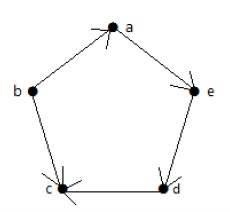The indegree and outdegree of other vertices are shown in the following table −

Vertex Indegree Outdegree
a 1 1
b 0 2
c 2 0
d 1 1
e 1 1

### Pendent Vertex

By using degree of a vertex, we have a two special types of vertices. A vertex with degree one is called a pendent vertex.

Example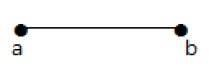Here, in this example, vertex ‘a’ and vertex ‘b’ have a connected edge ‘ab’. So with respect to the vertex ‘a’, there is only one edge towards vertex ‘b’ and similarly with respect to the vertex ‘b’, there is only one edge towards vertex ‘a’. Finally, vertex ‘a’ and vertex ‘b’ has degree as one which are also called as the pendent vertex.

### Isolated Vertex

A vertex with degree zero is called an isolated vertex.

Example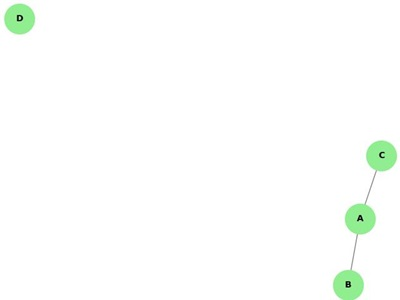Here, the vertex ‘a’ and vertex ‘b’ has a no connectivity between each other and also to any other vertices. So the degree of both the vertices ‘a’ and ‘b’ are zero. These are also called as isolated vertices.

Here are the norms of adjacency −

• In a graph, two vertices are said to be adjacent, if there is an edge between the two vertices. Here, the adjacency of vertices is maintained by the single edge that is connecting those two vertices.

• In a graph, two edges are said to be adjacent, if there is a common vertex between the two edges. Here, the adjacency of edges is maintained by the single vertex that is connecting two edges.

Example 1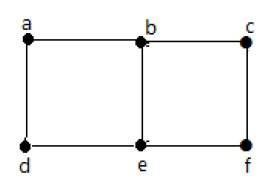In the above graph −

• ‘a’ and ‘b’ are the adjacent vertices, as there is a common edge ‘ab’ between them.

• ‘a’ and ‘d’ are the adjacent vertices, as there is a common edge ‘ad’ between them.

• ab’ and ‘be’ are the adjacent edges, as there is a common vertex ‘b’ between them.

• be’ and ‘de’ are the adjacent edges, as there is a common vertex ‘e’ between them.

Example 2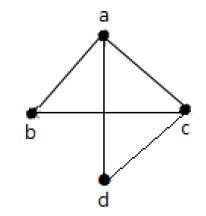In the above graph −

• ‘a’ and ‘d’ are the adjacent vertices, as there is a common edge ‘ad’ between them.

• ‘c’ and ‘b’ are the adjacent vertices, as there is a common edge ‘cb’ between them.

• ‘ad’ and ‘cd’ are the adjacent edges, as there is a common vertex ‘d’ between them.

• ‘ac’ and ‘cd’ are the adjacent edges, as there is a common vertex ‘c’ between them.

### Parallel Edges

In a graph, if a pair of vertices is connected by more than one edge, then those edges are called parallel edges.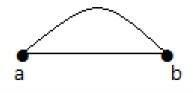In the above graph, ‘a’ and ‘b’ are the two vertices which are connected by two edges ‘ab’ and ‘ab’ between them. So it is called as a parallel edge.

### Multi Graph

A graph having parallel edges is known as a Multigraph.

Example 1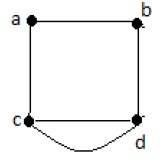In the above graph, there are five edges ‘ab’, ‘ac’, ‘cd’, ‘cd’, and ‘bd’. Since ‘c’ and ‘d’ have two parallel edges between them, it a Multigraph.

Example 2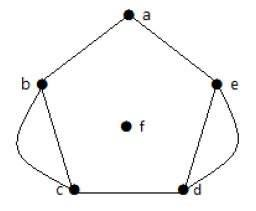In the above graph, the vertices ‘b’ and ‘c’ have two edges. The vertices ‘e’ and ‘d’ also have two edges between them. Hence it is a Multigraph.

### Degree Sequence of a Graph

If the degrees of all vertices in a graph are arranged in descending or ascending order, then the sequence obtained is known as the degree sequence of the graph.

Example 1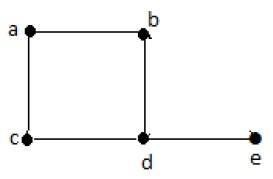Vertex Connecting to Degree A b c d e b,c a,d a,d c,b,e d 2 2 2 3 1

In the above graph, for the vertices {d, a, b, c, e}, the degree sequence is {3, 2, 2, 2, 1}.

Example 2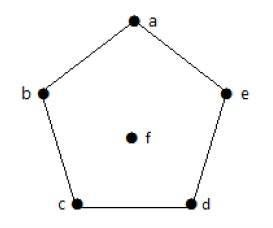Vertex Connecting to Degree A b c d e f b,e a,c b,d c,e a,d - 2 2 2 2 2 0

In the above graph, for the vertices {a, b, c, d, e, f}, the degree sequence is {2, 2, 2, 2, 2, 0}.